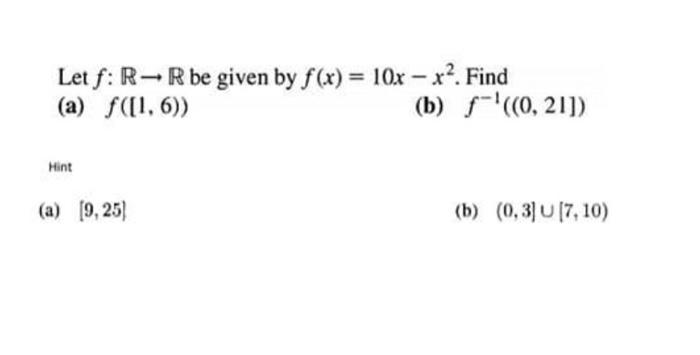### Create an Account

Already have account?

### Forgot Your Password ?

Home / Questions / Let f: R-Rbe given by f(x) = 10x - x?. Find (a) f([1, 6)) (b) f-'(0,21]) Mint (a) (9,25) (...

# Let f: R-Rbe given by f(x) = 10x - x?. Find (a) f([1, 6)) (b) f-'(0,21]) Mint (a) (9,25) (b) (0,3] 17, 10)

Let f: R-Rbe given by f(x) = 10x - x?. Find (a) f([1, 6)) (b) f-'(0,21]) Mint (a) (9,25) (b) (0,3] 17, 10)Apr 14 2021 View more View Less

#### Answer (Solved)Subscribe To Get Solution